RELATIVE ATOMIC MASS THE MOLE ATOMIC MASS Mass

• Slides: 18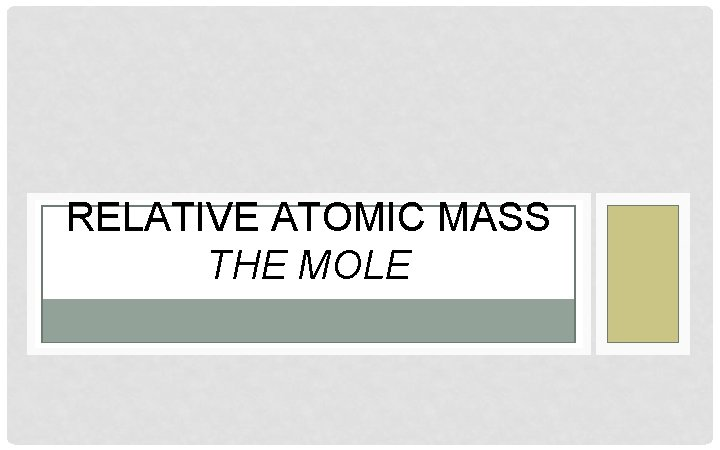RELATIVE ATOMIC MASS THE MOLEATOMIC MASS Mass: A measure of the amount of matter Atomic Mass: The average mass of all the isotopes of a particular element Atoms of different elements have different massesATOMIC MASS • The atomic mass is found by comparing the mass of an atom of an element to the mass of an atom of Carbon-12 • Carbon-12 is assigned an atomic mass of exactly 12. 00000000000 u (“u” stands for “unified atomic mass unit”)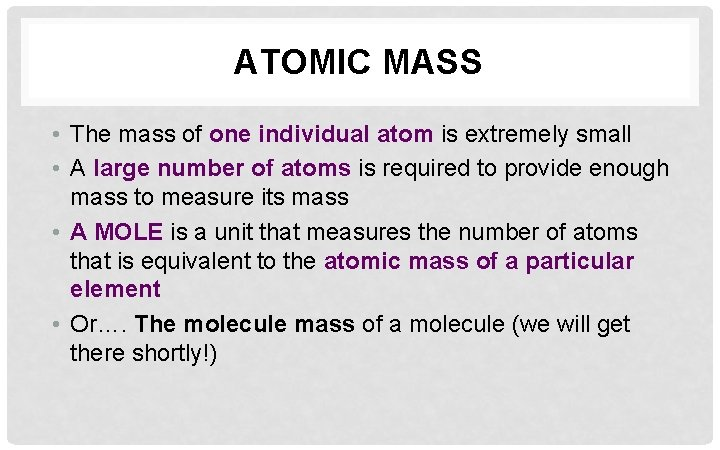ATOMIC MASS • The mass of one individual atom is extremely small • A large number of atoms is required to provide enough mass to measure its mass • A MOLE is a unit that measures the number of atoms that is equivalent to the atomic mass of a particular element • Or…. The molecule mass of a molecule (we will get there shortly!)INQUIRY QUESTIONS Form groups of 3 -4, collect a whiteboard and pens and answer following questions 1. Why does a mole of Carbon -12 weigh EXACTLY 12. 0000 g? 2. Why was carbon the first element assigned a weight? 3. When you look at the periodic table, why do elements have atomic masses with decimals? ?INQUIRY QUESTIONS Form groups of 3 -4, collect a whiteboard and pens and answer following questions 1. Why does a mole of Carbon -12 weigh EXACTLY 12. 0000 g? Actually, it’s the other way round, Avogadro wanted a mole to be EXACTLY 12. 000000 g of carbon. He picked 12. 000000 g, because carbon contains 6 protons and 6 neutrons in it’s nucleus.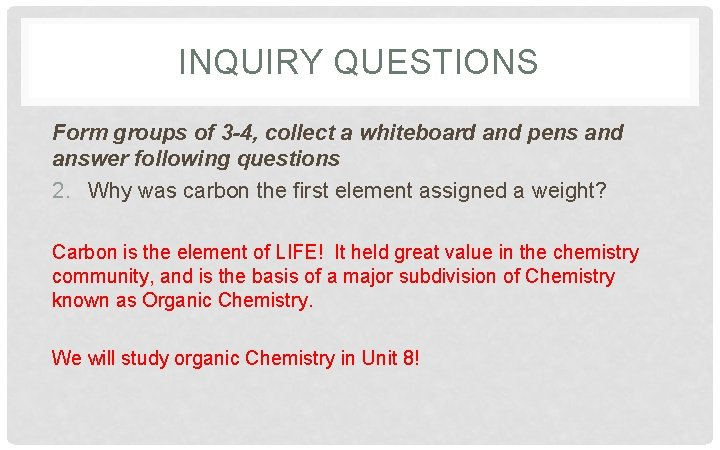INQUIRY QUESTIONS Form groups of 3 -4, collect a whiteboard and pens and answer following questions 2. Why was carbon the first element assigned a weight? Carbon is the element of LIFE! It held great value in the chemistry community, and is the basis of a major subdivision of Chemistry known as Organic Chemistry. We will study organic Chemistry in Unit 8!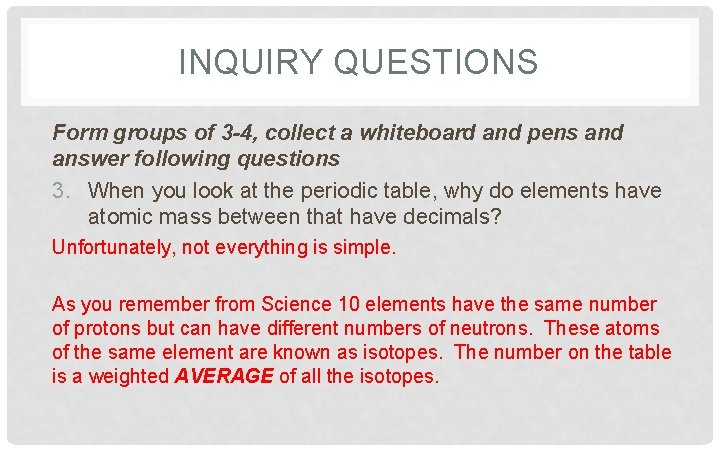INQUIRY QUESTIONS Form groups of 3 -4, collect a whiteboard and pens and answer following questions 3. When you look at the periodic table, why do elements have atomic mass between that have decimals? Unfortunately, not everything is simple. As you remember from Science 10 elements have the same number of protons but can have different numbers of neutrons. These atoms of the same element are known as isotopes. The number on the table is a weighted AVERAGE of all the isotopes.WHAT IS A UNIT? • Definitive magnitude • Examples: • 1 dozen = 12 items • 1 minute = 60 seconds • 1 year = 365 days Sig Figs are infinite!WHAT IS A MOLE? • Avogadro’s Number • 6. 022 141 x 1023 items per mole • The abbreviation for the unit mole is mol. (do not confuse this with molecules!)A MOLE OF PENNIES 1. Please collect the handout from the front of the room 2. Complete the activity with 1 or 2 neighbours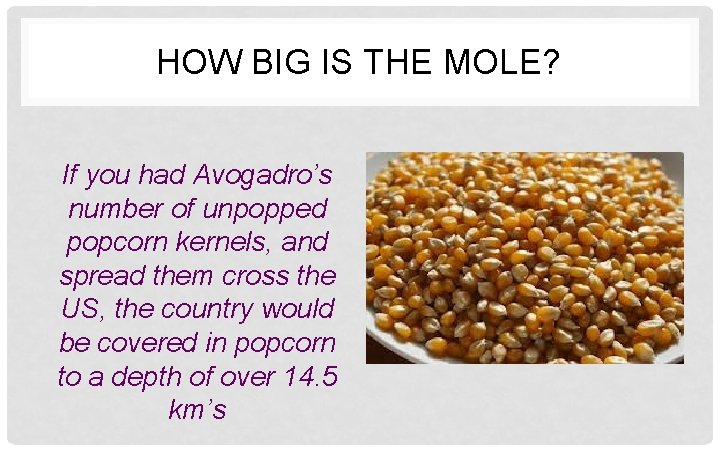HOW BIG IS THE MOLE? If you had Avogadro’s number of unpopped popcorn kernels, and spread them cross the US, the country would be covered in popcorn to a depth of over 14. 5 km’s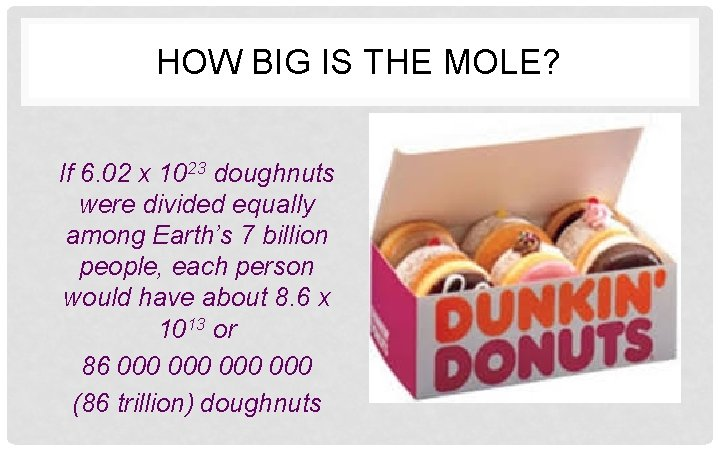HOW BIG IS THE MOLE? If 6. 02 x 1023 doughnuts were divided equally among Earth’s 7 billion people, each person would have about 8. 6 x 1013 or 86 000 000 (86 trillion) doughnutsHOW BIG IS THE MOLE? One mole of paper would make a stack that would reach to the moon more than 80 billion times.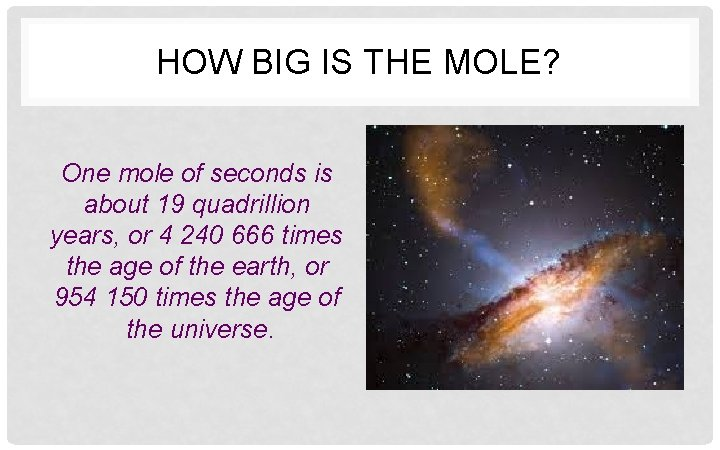HOW BIG IS THE MOLE? One mole of seconds is about 19 quadrillion years, or 4 240 666 times the age of the earth, or 954 150 times the age of the universe.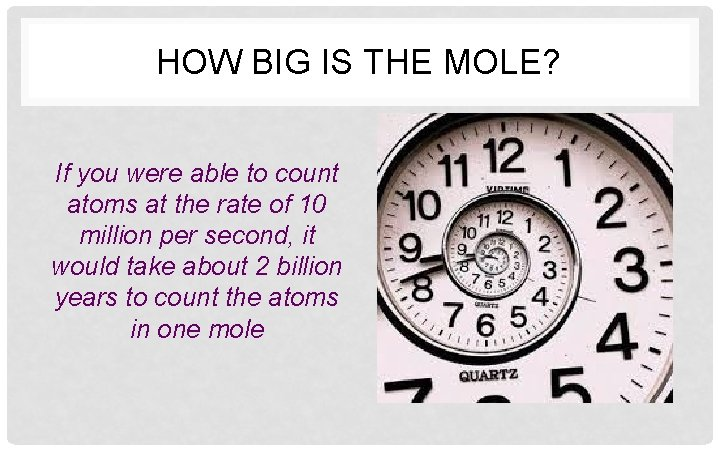HOW BIG IS THE MOLE? If you were able to count atoms at the rate of 10 million per second, it would take about 2 billion years to count the atoms in one mole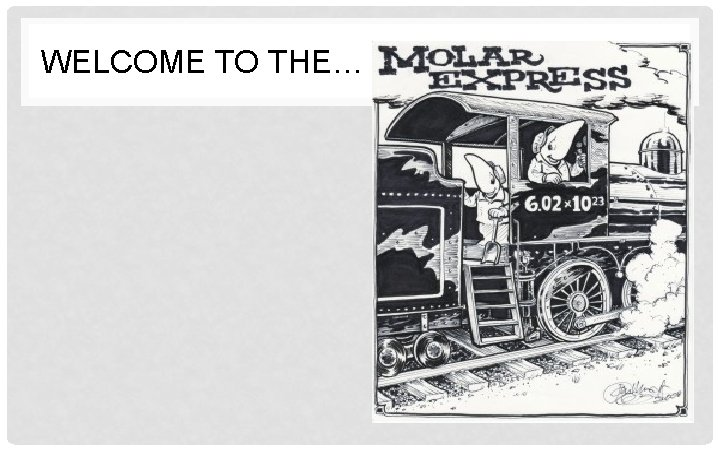WELCOME TO THE…HOW MANY LITHIUM ATOMS ARE IN 3. 2 MOL OF LITHIUM?